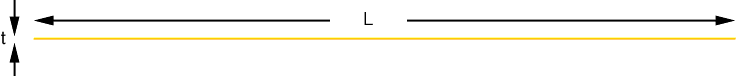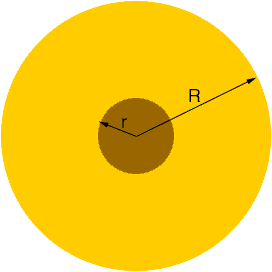Quandaries and Queries How would you calculate a diameter of a paper reel when the paper is 0,04 millimeters thick and for example 5000 meters of it is winded on a 76 mm (3inch) core. Regards Tuomas Tuomas, Think of the paper laid out as one long piece. The edge of this paper strip is a very long, thin rectangle of some length L and thickness t.This long, thin rectangle has area tL. Now roll the paper onto the reel and look at the end of the roll. Let R be its radius and r the radius of the core.The edge of the paper in now in the shape of a disk of radius R with a hole in its center of radius r. The area of this region isR2 -r2 =(R2 - r2) But the area of the edge of the paper has not changed, only its shape has changed. Hence tL =(R2 - r2) Penny Go to Math Central